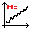#### File Exchange > Data Analysis >    Hurst ExponentAuthor:
OriginLab Technical Support
2/1/2016
Last Update:
6/15/2022
107
Total Ratings:
5
File Size:
23 KB
Average Rating:File Name:
HurstExponent.opx
File Version:
1.00
Minimum Versions:
Free
Summary:

Calculate Hurst exponent with rescaled range analysis.

Screen Shot and Video:
Description:

Purpose:

The tool can be used to:

• Calculate classical Hurst exponents (R/S) and corrected Hurst exponents (R/S-AL).
• Show a Log-Log plot of R/S statistics vs subseries length.

Installation:

Download the file HurstExponent.opx, and then drag-and-drop onto the Origin workspace. An icon will appear in the Apps Gallery window.

Operation:

1. Highlight a column of time series data in the worksheet.
2. Click the Hurst Exponent icon in the Apps Gallery window.
3. In the dialog box that opens:
1. Set Start Index and End Index to define partial series.
2. Set Minimum Subseries Length to define the minimum length of subseries when partitioning the time series. The default value is 50.
When a trend or seasonality exists in time series data, minimum subseries should exhibit the time series pattern (e.g. for seasonal data, Minimum Subseries Length should be no less than the length of the period).
3. Check the Log-Log Plot to output the plot.
4. Click the OK button to output a worksheet with results and a Log-Log plot.

Algorithm:
$$(R/S)_n = \frac{1}{d} \displaystyle \sum R_m/S_m$$
where input time series is divided into d subseries of length n, $$R_m$$and$$S_m$$are the range and standard deviation in each subseries.
$$E(R/S)_n = \begin{cases} \frac{ (n-0.5) \Gamma(\frac{n-1}{2}) }{n \sqrt{ \pi } \Gamma( \frac{n}{2} ) } \displaystyle \sum_{i=1}^{n-1} \sqrt{ \frac{n-i}{i} } &\text{for} \; n \leq 340, \\ \frac{ n-0.5 }{n \sqrt{ \frac{n \pi}{2} } } \displaystyle \sum_{i=1}^{n-1} \sqrt{ \frac{n-i}{i} } &\text{for} \; n > 340 \end{cases}$$
$$R/S-AL = (R/S)_n - E(R/S)_n + \sqrt{ \frac{ n \pi }{2}}$$
Slope of $$(R/S)_n$$ vs. n in Log-Log plot is the classic Hurst exponent, and slope of R/S-AL vs. n in Log-Log plot is the AL corrected Hurst exponent.

Reference:

1. R. Weron (2002) Estimating long range dependence: finite sample properties and confidence intervals, Physica A 312, 285-299.

 06/07/2022 OriginLab Hi kobrinskyevgeny, I replied you in the email for methods used in the app. We will add Algorithm and Reference sections in this page. Thanks. 06/06/2022 kobrinskyevgeny Could you please tell what methods were used to calculate H exponent? Thank you.11/06/2017 luocaiwei1212 test04/17/2017 fermath very good job05/29/2016 fabiobreunig